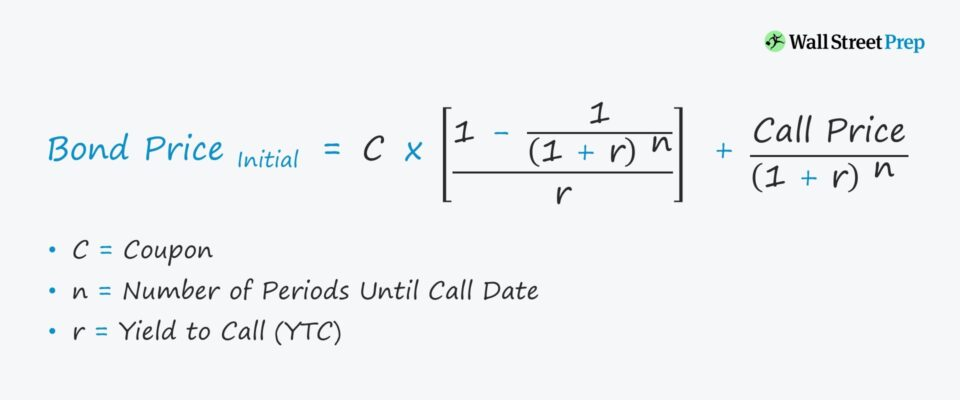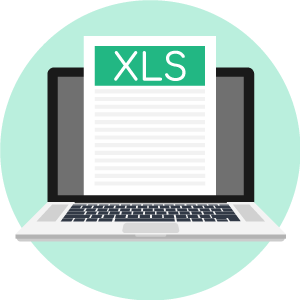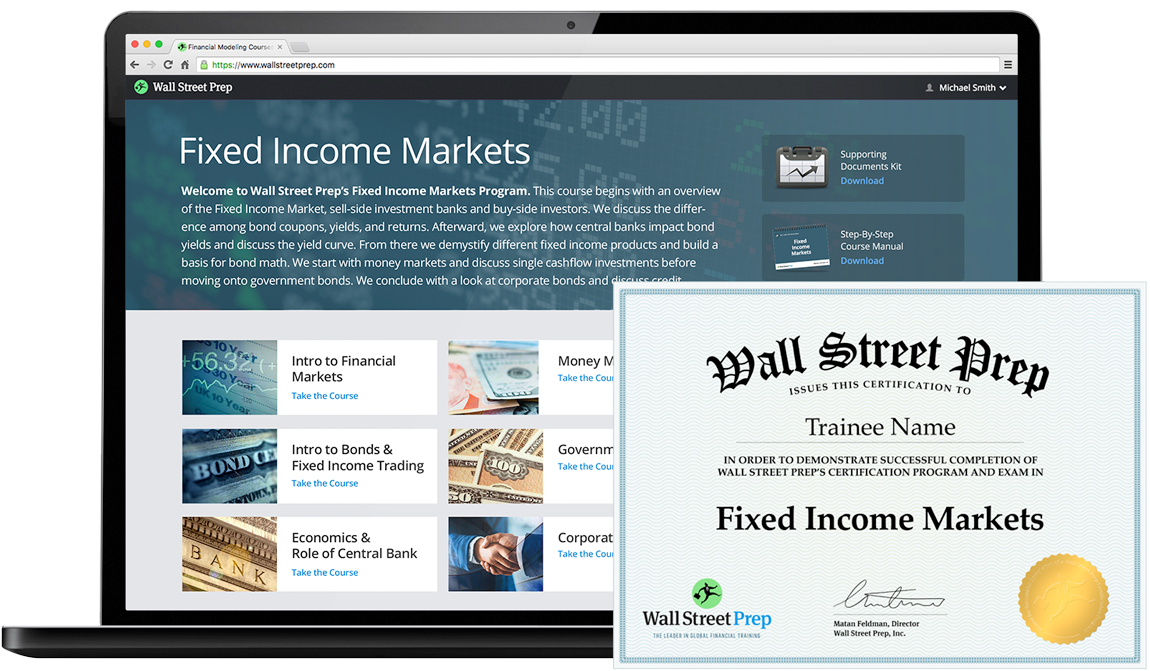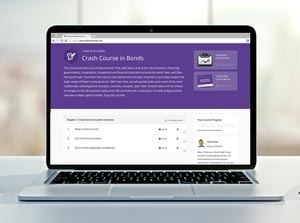# Yield to Call (YTC)

Understand the Yield to Call (YTC) Concept• What is the definition of yield to call (YTC)?
• Which formula calculates yield to call (YTC)?
• How is yield to call (YTC) different from yield to maturity (YTM)?
• How can the yield to call (YTC) on a bond be calculated in Excel?

## Yield to Call (YTC) Definition

If a callable bond is redeemed at the next call date – as opposed to the original maturity date – then the return is the yield to call (YTC).

The yield to call (YTC) metric implies that a callable bond was redeemed (i.e. paid off) sooner than the stated maturity date.

If a bond issuance is callable, then the issuer can redeem (i.e. retire) the borrowing prior to maturity.

Most often, the reason behind an issuer calling a bond early is to:

• Refinance in a Low Interest Rate Environment (or)
• Reduce the Debt % in the Capital Structure

Callable bonds provide the issuer with the option to pay off a portion or all of the debt obligation, with a schedule that clearly outlines when prepayment is permitted.

For instance, if a bond’s call protection is abbreviated as “NC/2”, that means the bond is not allowed to be redeemed within the next two years.

Beyond the stated non-callable period, the bonds can be retired earlier than maturity, typically presented in a schedule with more than one call date listed.

Side Note: Hypothetically, the yield to call (YTC) can be calculated as if the bond was redeemed on a date later than the first call date, but most YTCs are calculated based on redemption on the earliest date possible.

###### Callable Bonds

The fixed call price is usually set at a minor premium above the face (par) value – a common feature included for callable bonds to make them more attractive to risk-averse investors.

Additionally, the call provision results in prepayment fees, which are also intended to make the bond offering more marketable.

All else being equal, bonds with a callable provision should exhibit higher yields than comparable, non-callable bonds.

## Yield to Call (YTC) Formula

Given the pricing data, coupon rate, years until maturity, and face value on a bond, it is possible to estimate the yield to call (YTC) by trial-and-error.

However, the more common approach is to use either Excel or a financial calculator.

The formula below calculates the interest rate that sets the present value (PV) of a bond’s scheduled coupon payments and the call price equal to the current bond price.

### Bond Price Formula

• Initial Bond Price (PV) = C × [1 – {1 / (1 + r) ^ n} / r] + Call Price / (1 + r) ^ n

Where:

• C = Coupon
• r = Yield to Call
• n = Number of Periods Until Call Date

Note that the convention on each input must match for the formula to work (i.e. the bond quote vs bond price, call price vs payment on call date).

For example, let’s assume a bond becomes callable in 1 year (i.e. “NC/1”) with the following characteristics:

• Par Value (FV) = 100
• Coupon Rate = 8%
• Coupon = 100 × 8% = 8
• Call Price = 104
• Number of Periods (n) = 1
• Yield to Call = 6.7%

If we enter these assumptions into our formula, the initial bond price (PV) comes out to 105.

• Initial Bond Price (PV) = 8 × {1 – [1 / (1 + 6.7%) ^ 1] / 6.7%} + 104 / (1 + 6.7%) ^ 1
• Initial Bond Price (PV) = 105

## Yield to Call (YTC) vs Yield to Maturity (YTM)

Generally, the purpose of calculating the yield to call (YTC) is to compare it to the yield to maturity (YTM).

• If YTC > YTM → Redeem
• If YTM > YTC → Hold Until Maturity

More specifically, the lowest possible return – other than if the issuer were to default – is referred to as the yield to worst (YTM), which helps bondholders determine the chance of an issuer redeeming its bonds early.

If the yield to call (YTC) is greater than the yield to maturity (YTM), it is reasonable to assume there is a high risk that the bonds are unlikely to remain trading until maturity.

Hence, the yield to worst (YTW) is most applicable when a callable bond is trading at a premium to par.

## Yield to Call (YTC) Calculator – Excel Template

We’ll now move to a modeling exercise, which you can access by filling out the form below.Submitting ...

## Yield to Call (YTC) Example Calculation

In our illustrative example, we’ll calculate the yield to call (YTC) on a ten-year callable bond issuance that was finalized on 12/31/21.

• Settlement Date: 12/31/21
• Maturity Date: 12/31/31

Moreover, the bond becomes callable after four years, i.e. “NC/4”, and the call price carries a 3% premium over the par value (“100″).

The bond’s call price, denoted as “103,” is the price the issuer must pay to redeem the issuance prior to maturity.

• First Call Date: 12/31/25
• Call Price: 103

On the date of issuance, the par value of the bond (FV) was \$1,000 – but the current bond price (PV) is \$980 (“98”).

• Face Value of Bond (FV): \$1,000
• Current Bond Price (PV): \$980
• Bond Quote (% of Par): 98

The final set of assumptions is related to the coupon, in which the bond pays a semi-annual coupon at an annual interest rate of 8%.

• Frequency of Coupon: 2 (Semi-Annual)
• Annual Coupon Rate (%): 8%
• Annual Coupon: \$80

The yield to call (YTC) can now be calculated using the “YIELD” Excel function.

### Yield Excel Function

• Yield to Call (YTC) = “YIELD (settlement, maturity, rate, pr, redemption, frequency)”

Specific to the yield to call, “maturity” is set to the earliest call date while “redemption” is the call price.

• Yield to Call (YTC) = “YIELD (12/31/21, 12/31/25, 8%, 98, 103, 2)”

The yield to call (YTC) on our bond is 9.25%, as shown by the screenshot of our model below.Globally Recognized Certification Program

#### Get the Fixed Income Markets Certification (FIMC©)

Wall Street Prep's globally recognized certification program prepares trainees with the skills they need to succeed as a Fixed Income Trader on either the Buy Side or Sell Side.Inline FeedbacksLearn Online: Crash Course in Bonds

For those pursuing fixed income research, investments, sales and trading or investment banking.

X

The Wall Street Prep Quicklesson Series

7 Free Financial Modeling Lessons

Get instant access to video lessons taught by experienced investment bankers. Learn financial statement modeling, DCF, M&A, LBO, Comps and Excel shortcuts.